# Fermat's last theorem

(diff) ← Older revision | Latest revision (diff) | Newer revision → (diff)

Fermat's last theorem surely was mathematics' most celebrated and notorious open problem. Its investigation sparked fundamental advances in the mathematical sciences.

Fermat's last theorem is the claim thathas no solutions in non-zero integers for. Forthere is an infinity of solutions, such as: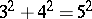;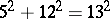;. These are called the Pythagorean triples, in view of the Pythagoras theorem. However, around 1637, the French jurist P. de Fermat wrote in the margin of his copy of the Arithmetika of Diophantus that: "Cubum autem in duos cubos, aut quadrato-quadratum in duos quadrato-quadratos, et generaliter nullam in infinitum ultra quadratum potestatem in duos ejusdem nominis fas est dividere; cujus rei demonstrationem mirabilem sane detexi. Hanc marginis exiguitas non caperet" .

(That is, "to split a cube into two cubes, or a fourth (biquadratic) power into two fourth powers, or indeed any higher power unto infinity into two like powers, is impossible; and I have a marvelous proof for this. But the margin is too narrow to contain it" .)

On Fermat's death in 1665, his son Samuel proceeded to collect Fermat's mathematical correspondence. That, and a reprint of the Arithmetika, together with Fermat's marginal notes, was published in 1670. By the end of the eighteenth century all of Fermat's other remarks had been dealt with, one way or the other: either properly proved or shown to be false. Only this one remark remained, hence the name "last theorem" .

Of course, one need only deal with the exponents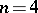and, an odd prime number. In one of the few actual proofs he left behind, Fermat himself had dealt with the case. It was many years later, in 1753, that L. Euler dealt with the case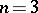. There was an alleged omission in the argument, later filled in by C.F. Gauss. P.G.L. Dirichlet and A.M. Legendre proved the casein 1825 and G. Lamé settled the case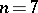in 1839; Dirichlet had proved the casein 1832.

It is quite absurd to suppose that Fermat had a proof of the "last theorem" , or that he even continued to believe that he had one much beyond the moment he scribbled the claim in that notorious margin. Other than for the exponentsand, which were within his reach, Fermat never boasted about the matter in his correspondence in the thirty or so remaining years of his life.

For symmetry, set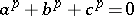, withan odd prime number. It is now easy to show that if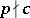, then there is an integerso that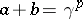, whilst if, then. Anyway, the cases(the so-called first case),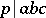(the second case) are rather different. N.H. Abel used such formulas to show that if in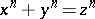, withand, one of,, oris some prime power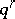, then the exponentmust be prime number, the prime power must be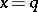,differs fromby, anddivides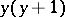. Similarly, S. Germain, generalized by A.M. Legendre, showed that there is no solution for the first case if bothand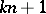are prime; heremay take various small values. It followed that there is no solution for the first case when the exponentis less than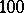.

Of course,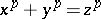equals, where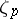is a primitiveth root of unity. Thus each of the principal ideals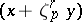is theth power of an ideal of the cyclotomic field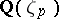; indeed, of a principal ideal if the class number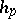of that field is prime to. E.E. Kummer proved Fermat's last theorem for such regular prime numbers in 1847. His researches provided a seemingly straightforward characterization of regularity in terms of the behaviour of the Bernoulli numbers modulo; his study of cyclotomic number fields initiated the subject now known as algebraic number theory, and by dealing with the primes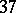,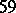and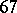, which are only "a little irregular" , Kummer proved Fermat's last theorem beyond exponent(up to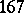, where the calculations became just to burdensome).

The next 120 years see surprisingly little fundamental advance on Kummer's contributions to Fermat's last theorem. H. Vandiver corrected part of Kummer's work and refined his criteria for irregularity. By 1993, further such refinements and ingenious computer computations had settled Fermat's last theorem for exponents up to four million.

At the turn of the century, work relying on a complicated analysis of Kummer's conditions led to the criterion that if there is to be a solution to the first case for exponent, thenmust divide the Fermat quotients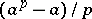for both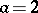and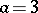. However, (one now knows that) the only prime numbers up to, say, satisfying the criterion forare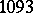and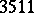, whilst for basethese are just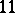and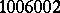. By 1993, the first case criterion had been proved to be necessary for all bases up to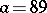, settling that case beyond exponent.

By applying techniques from transcendental number theory and Diophantine approximation, K. Inkeri had shown that a putative solution in the case of exponentwould have, say, larger than about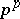. Thus, by 1993 it was known that one could not state any counterexample to Fermat's claim, for if there were one it would be millions of digits long.

Consider the cubic curve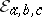given by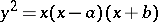, where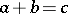in non-zero integers. This Frey curveis an elliptic curve with naive discriminant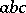. In 1985 G. Frey had remarked that, for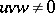and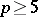, ifand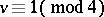, then, remarkably, the curve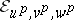is semi-stable and its supposed existence seemed likely to contradict the Taniyama–Shimura modularity conjecture for elliptic curves over the rational number field. In the language of the 1970s,could apparently not be a Weil curve. A suggestion of J.-P. Serre, his so-called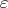-conjecture, was proved by K. Ribet in 1986, entailing that indeed the truth of the modularity conjecture, and then just for semi-stable elliptic curves, implies Fermat's last theorem. At the time, though, it was believed that the modularity conjecture was inaccessible; that its proof was at least a generation away.

It was therefore an enormous surprise and excitement when, at Cambridge University in 1993, A. Wiles announced he had proved Fermat's last theorem by establishing the modularity conjecture for semi-stable elliptic curves over. There followed a year or so of alarms in which a subtle gap in the argument had to be filled. In 1995, a paper of Wiles, augmented by a further joint note with R. Taylor, finally proved Fermat's allegation [a5].

Gauss had dismissed Fermat's equation as just one of a multitude of such Diophantine equations, and Kummer had made it clear that he valued his work on the higher reciprocity laws far more than its eventual application to Fermat's last theorem. Fittingly, Fermat's last theorem is now a corollary of far more fundamental results.

For the legends see [a1]. Kummer's work is detailed in [a2], whilst [a3] gives near complete references to work on Fermat's last theorem up to the 1970s. Finally, [a4] provides an accessible introduction to Wiles' proof "in an amusing and intriguing collection of tidbits, anecdotes, footnotes, exercises, references, illustrations, and more" .

How to Cite This Entry:
Fermat's last theorem. Encyclopedia of Mathematics. URL: http://encyclopediaofmath.org/index.php?title=Fermat%27s_last_theorem&oldid=18735
This article was adapted from an original article by A.J. van der Poorten (originator), which appeared in Encyclopedia of Mathematics - ISBN 1402006098. See original article